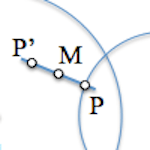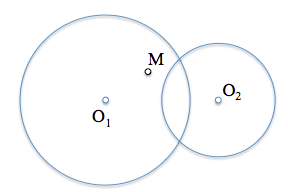# Determinación de un segmento conocido su punto medio [Statement]An interesting metric geometry problem that can enlighten the way to find solutions is to determine a segment of known its midpoint with additional restrictions.

And that a segment is determined by its ends (colon), in plane need four values (simple data) to set their Cartesian coordinates.

Knowledge of its midpoint “M” supposed to impose two restrictions (point coordinates) let us two degrees of freedom to determine the set of solutions. Therefore impose two new constraints to restrict solutions to a finite number of these, such as to ensure that their ends rest on two circles coplanar.

The problem statement could be therefore:

Determine the segments that are supported on two circles and having at point M as the midpoint.The conditions of step to the ends of the segment does not influence the overall model resolution, as discussed by posing the problem analysis.

The figure shows the two circles on which must rest the segment, and its midpoint M.

• What would change the problem if one of the circles were a straight?
• What if they were two lines?
• How many solutions does the problem?

The problem is left open to the reader's analysis. You can view the solution, once published, in the following link:

Solution segment known its midpoint

Metric Geometry